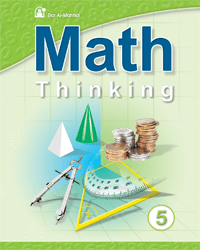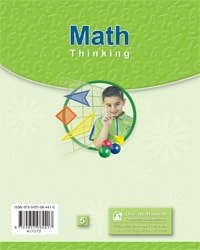+962 (6) 5533889
info@manhal.com
+962 (78) 7000522
support@manhal.com

# Math Thinking Level 05 book

• Book
• Description
• Related
• Rating
(0)
••6650
0
0
0
• Math Thinking 05 is one of Dar Al Manhal educational books that comes within Math Thinking curriculum which includes 6 books that teach kids mathematics basics in English language.

The book, Math Thinking 05 is divided into twelve units which include numbers of 9 digits (millions), relations between them,  and addition and subtraction within.

The book, Math Thinking 05, also teaches multiplication and division with three numbers, and the lowest and the greatest common divisor, in addition to fractions, decimal fractions, number theory, and calculating the circumference, area, surface and volume, probability and statistics.

The book, Math Thinking 05, also includes solving mathematical problems of each unit to test what kids have learned from it.

150
Soft Cover
Product Dimensions and Weight
21 cm
28 cm
435 Gm
2005/10/2419
5
One
\$ 8.8
• Math
• Ammar Dkeidek And Others
• 2005
• English
• 9-11 Year
x
\$220